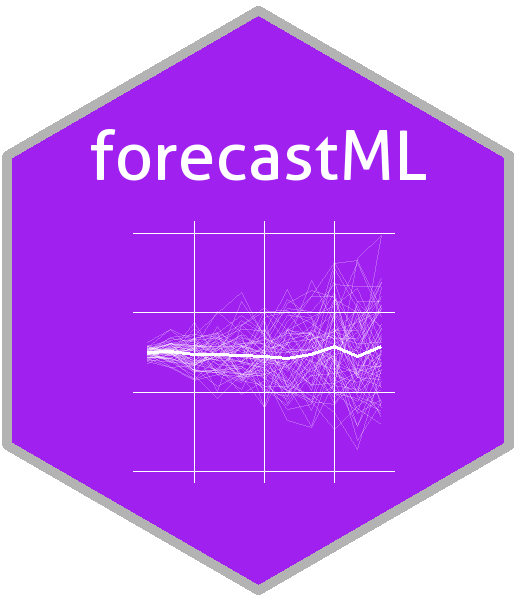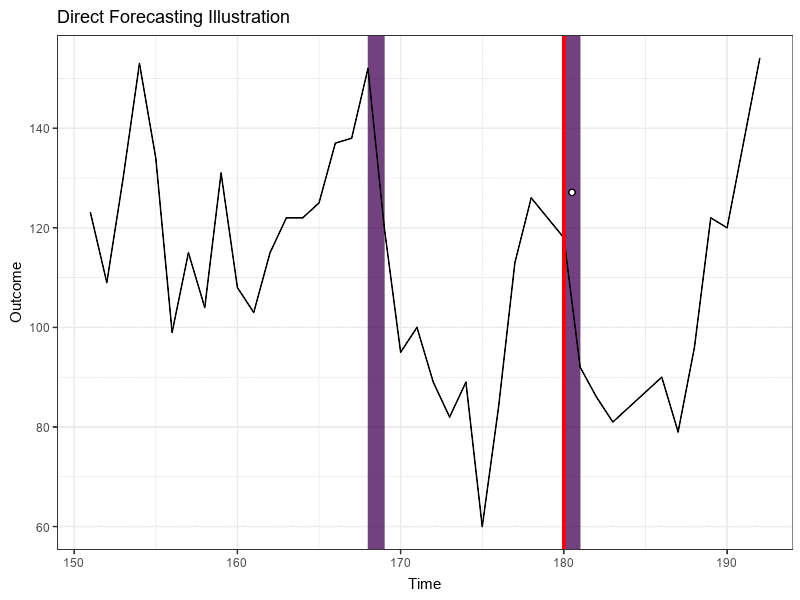# forecastML Overview

#### 2020-01-06# Purpose

The purpose of `forecastML` is to provide a series of functions and visualizations that simplify the process of multi-step-ahead direct forecasting with standard machine learning algorithms. It’s a wrapper package aimed at providing maximum flexibility in model-building–choose any machine learning algorithm from any `R` or `Python` package–while helping the user quickly assess the (a) accuracy, (b) stability, and (c) generalizability of grouped (i.e., multiple related time series) and ungrouped single-outcome forecasts produced from potentially high-dimensional modeling datasets.

This package is inspired by Bergmeir, Hyndman, and Koo’s 2018 paper A note on the validity of cross-validation for evaluating autoregressive time series prediction. In particular, `forecastML` makes use of

• lagged, grouped, dynamic, and static features,
• simple wrapper functions that support models from any `R` or `Python` package,
• nested cross-validation with (a) user-specified standard cross-validation in the inner loop and (b) block-contiguous validation datasets in the outer loop, and
• parallel processing with the `future` package

to build and evaluate high-dimensional forecast models without having to use methods that are time series specific.

The following quote from Bergmeir et al.’s article nicely sums up the aim of this package:

“When purely (non-linear, nonparametric) autoregressive methods are applied to forecasting problems, as is often the case (e.g., when using Machine Learning methods), the aforementioned problems of CV are largely irrelevant, and CV can and should be used without modification, as in the independent case.”

# Direct Forecasting

In contrast to the recursive or iterated method for producing multi-step-ahead forecasts used in traditional forecasting methods like ARIMA, direct forecasting involves creating a series of distinct horizon-specific models. Though several hybrid methods exist for producing multi-step forecasts, the simple direct forecasting method with used in `forecastML` lets us avoid the exponentially more difficult problem of having to “predict the predictors” for forecast horizons beyond 1-step-ahead.

Below are some resources for learning more about multi-step forecasting strategies:

The animation below shows how historical data is used to create a 1-to-12-step-ahead forecast for a 12-step-horizon forecast model using lagged predictors or features. Though feature lags greater than 12 steps can be used to make use of additional historical predictive information, a 12-step-horizon direct forecast model requires feature lags >= 12. This animation is roughly equivalent to how a 12-period seasonal ARIMA(0, 0, 0)(1, 0, 0) model uses historical data to produce forecasts.# Approach to Forecasting

The forecasting approach used in `forecastML` involves the following steps:

1. Build a series of horizon-specific short-, medium-, and long-term forecast models.

2. Assess model generalization peformance across a variety of heldout datasets through time.

3. Select those models that consistently performed the best at each forecast horizon and combine them to produce a single ensemble forecast.

Below is a plot of 5 forecast models used to produce a single 12-step-ahead forecast where each color represents a distinct horizon-specific ML model. From left to right these models are:

1: A feed-forward neural network (purple); 2: An ensemble of ML models; 3: A boosted tree model; 4: A LASSO regression model; 5: A LASSO regression model (yellow).